# Floyd Warshall Algorithm (Python) | Dynamic Programming

In this article, we will study what is Floyd Warshall Algorithm in the field of Dynamic Programming. We will also study the example and the python code with its corresponding output to learn and understand the algorithm. At last, we will go through the practical real-world application of the Floyd Warshall Algorithm.

## What is Floyd Warshall Algorithm?

Just like Dijkstra’s algorithm, the Floyd Warshall algorithm is used to find the shortest path between all vertices in the weighted graph. This algorithm works with both directed and undirected graphs but it does not work along with the graph with negative cycles. Therefore, if the distance from the vertex v from itself is negative then we can assume that the graph has the presence of a negative cycle. This algorithm follows the dynamic programming approach as its working pattern. Here the algorithm doesn’t construct the path itself but it can reconstruct the path with a simple modification. Floyd Warshall algorithm is also known as Roy Warshall algorithm or Roy-Floyd algorithm. Let us study the working of the Floyd Warshall algorithm.

### Algorithm

We construct a matrix D that gives the length of the shortest path between each pair of nodes.

The algorithm initializes D to L, that is, to the direct distances between nodes. It then does n iterations, after iteration k, D gives the length of the shortest paths that only use nodes in {1,2….k} as intermediate nodes.

After n iterations, D, therefore, gives the length of shortest paths using any of the nodes in N as an intermediate node. If Dk represents the matrix D after kth iteration it can be implemented by

Dk [i , j] = min (Dk-1 [i , j], Dk-1 [i, k] + Dk-1 [k , j])

We use the principle of optimality to compute length from i to j passing through k.

## How Floyd Warshall Algorithm Works (Example)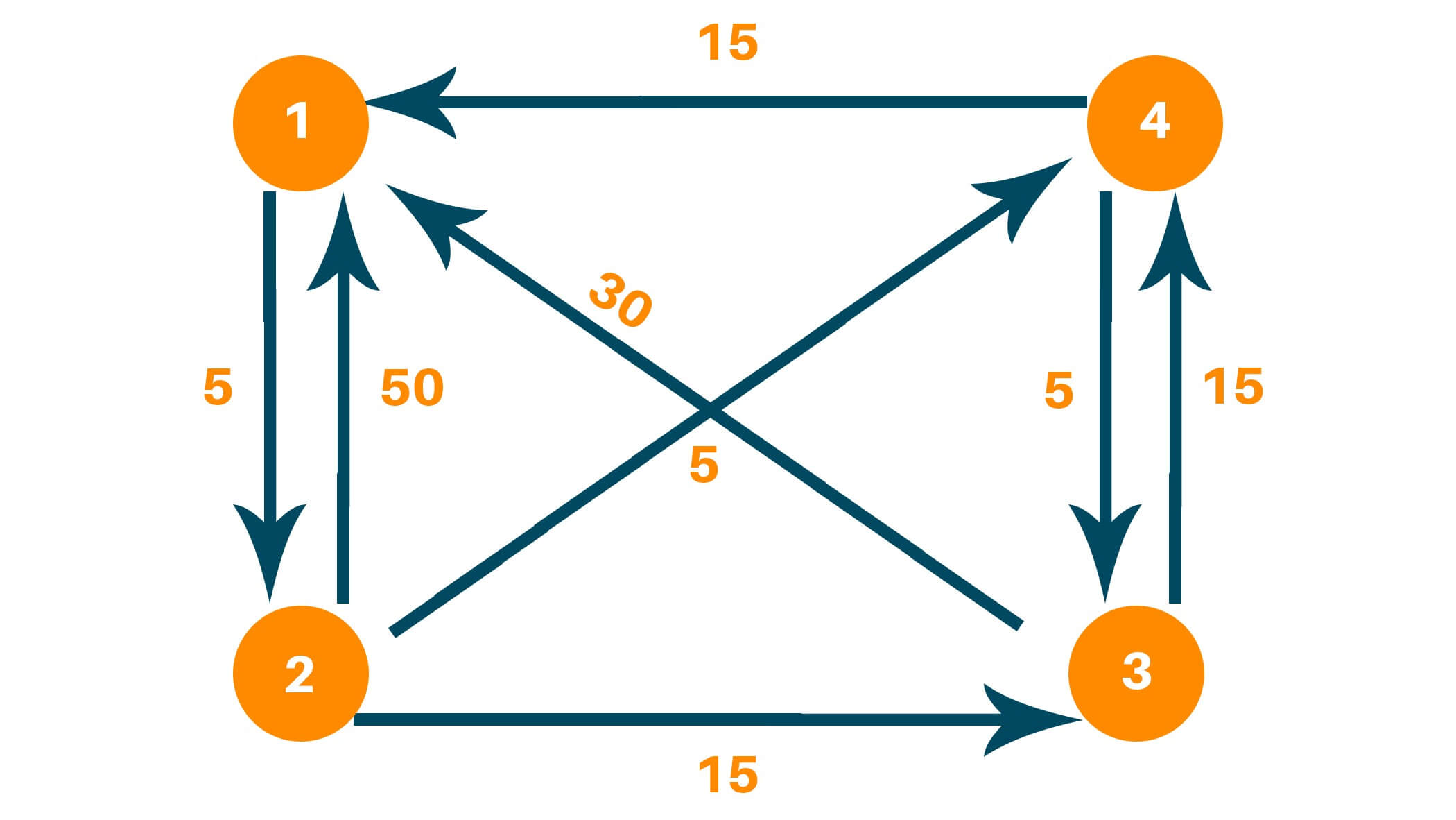Creating matrix D0 contains the distance between each node with ‘0’ as an intermediate node.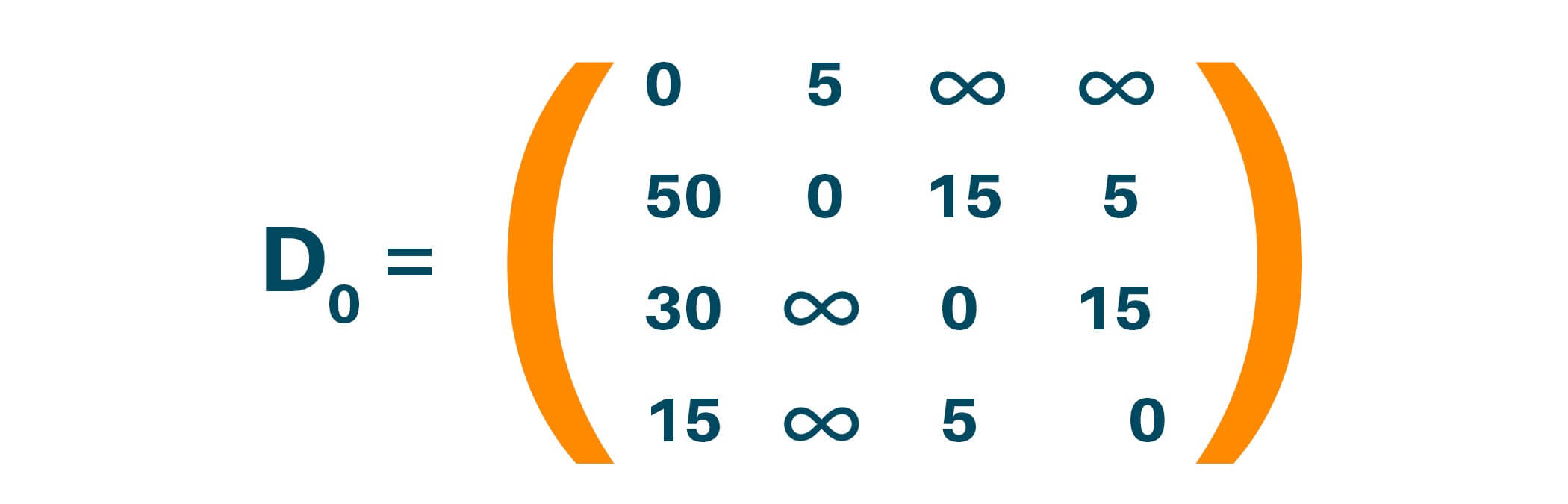Updating matrix D1 which contains the distance between each node with ‘1’ as an intermediate node. Update distance if minimum distance value smaller than existing distance value found.

Dk [i , j] = min (Dk-1 [i , j], Dk-1 [i, k] + Dk-1 [k , j])

D1[3, 2] = min(D1-1[3, 2], D1-1 [3, 1] + D1-1 [1 , 2])

D1[4, 2] = min(Infinity, 35)

Dk [i , j] = min (Dk-1 [i , j], Dk-1 [i, k] + Dk-1 [k , j])

D1[4, 2] = min(D1-1[4, 2], D1-1 [4, 1] + D1-1 [1 , 2])

D1[4, 2] = min(Infinity, 20)

Here distance (3, 2) is updated from infinity to 35, and distance (4, 2) is updated from infinity to 20 as shown below.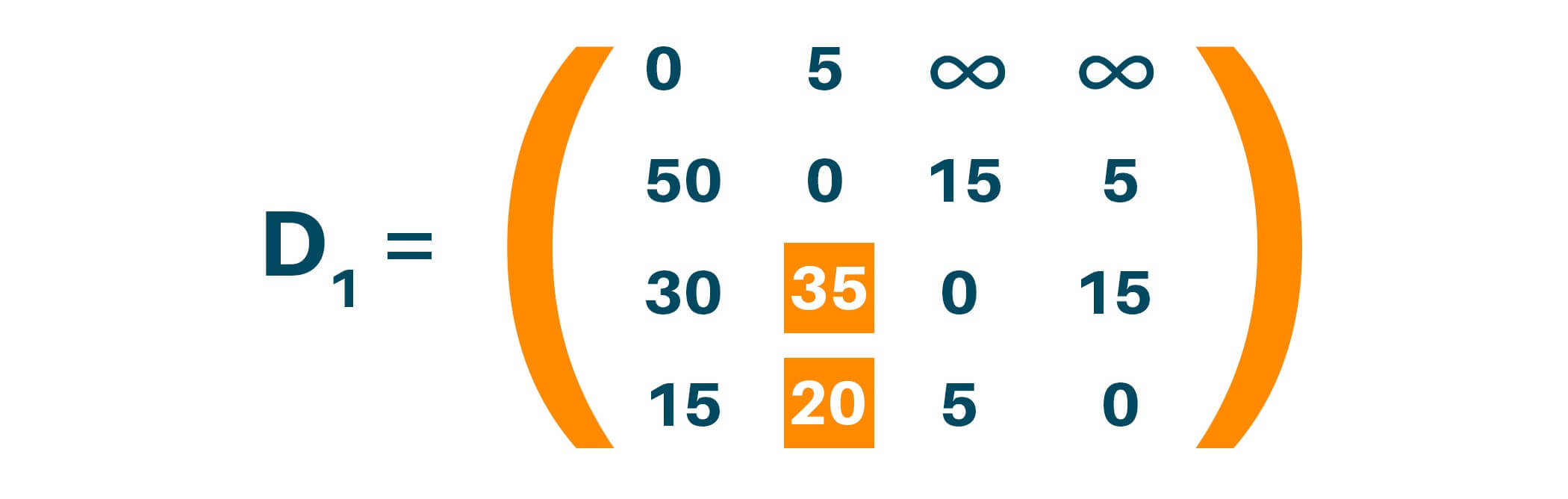Updating matrix D2 contains the distance between two nodes with ‘2’ as an intermediate node.

D2 [i , j] = min (Dk-1 [i , j], Dk-1 [i, k] + Dk-1 [k , j])

D2[1, 3] = min(D2-1[1, 3], D2-1 [1, 2] + D2-1 [2 , 3])

D2[1, 3] = min(Infinity, 20)

Dk [i , j] = min (Dk-1 [i , j], Dk-1 [i, k] + Dk-1 [k , j])

D2[1, 4] = min(D2-1[1, 4], D2-1 [1, 2] + D2-1 [2 , 4])

D2[1, 4] = min(Infinity, 10)

Update distance if minimum distance value smaller than existing distance value found. Here distance (1, 3) is updated from infinity to 20, and distance (1, 4) is updated from infinity to 10.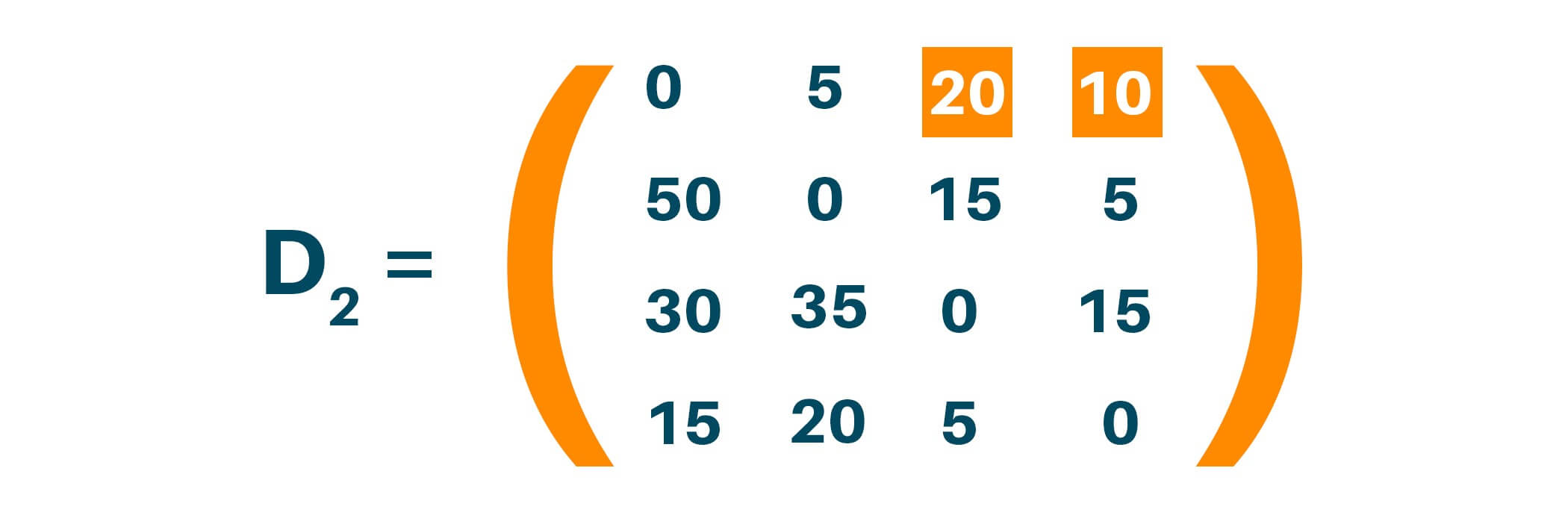Updating matrix D3  contains the distance between two nodes with ‘3’ as an intermediate node.

Dk [i , j] = min (Dk-1 [i , j], Dk-1 [i, k] + Dk-1 [k , j])

D3[2, 1] = min(D3-1[2, 1], D3-1 [2, 3] + D3-1 [3 , 1])

D3[2, 1] = min(50, 45)

Update distance if minimum distance value smaller than existing distance value found. Here distance (2, 1) is updated from 50 to 45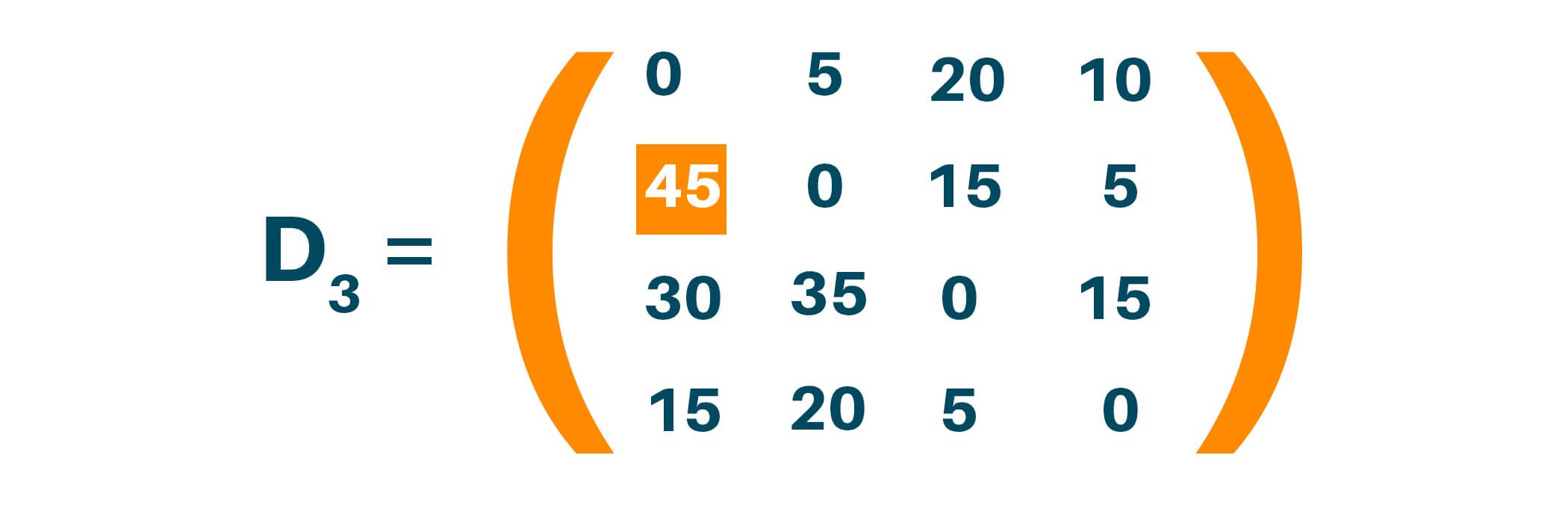Updating matrix D4 contains the distance between two nodes with ‘4’ as an intermediate node. Update distance if minimum distance value smaller than existing distance value found.

Dk [i , j] = min (Dk-1 [i , j], Dk-1 [i, k] + Dk-1 [k , j])

D4[1, 3] = min(D4-1[1, 3], D4-1 [1, 4] + D4-1 [4 , 3])

D4[1, 3] = min(20, 15)

Dk [i , j] = min (Dk-1 [i , j], Dk-1 [i, k] + Dk-1 [k , j])

D4[2, 1] = min(D4-1[2, 1], D4-1 [2, 4] + D4-1 [4 , 1])

D4[1, 3] = min(45, 20)

Dk [i , j] = min (Dk-1 [i , j], Dk-1 [i, k] + Dk-1 [k , j])

D4[2, 3] = min(D4-1[2, 3], D4-1 [2, 4] + D4-1 [4 , 3])

D4[2, 3] = min(15, 10)

Here distance (1, 3) is updated from 20 to 15; distance (2, 1) is updated from 45 to 20, and distance (2, 3) is updated from 15 to 10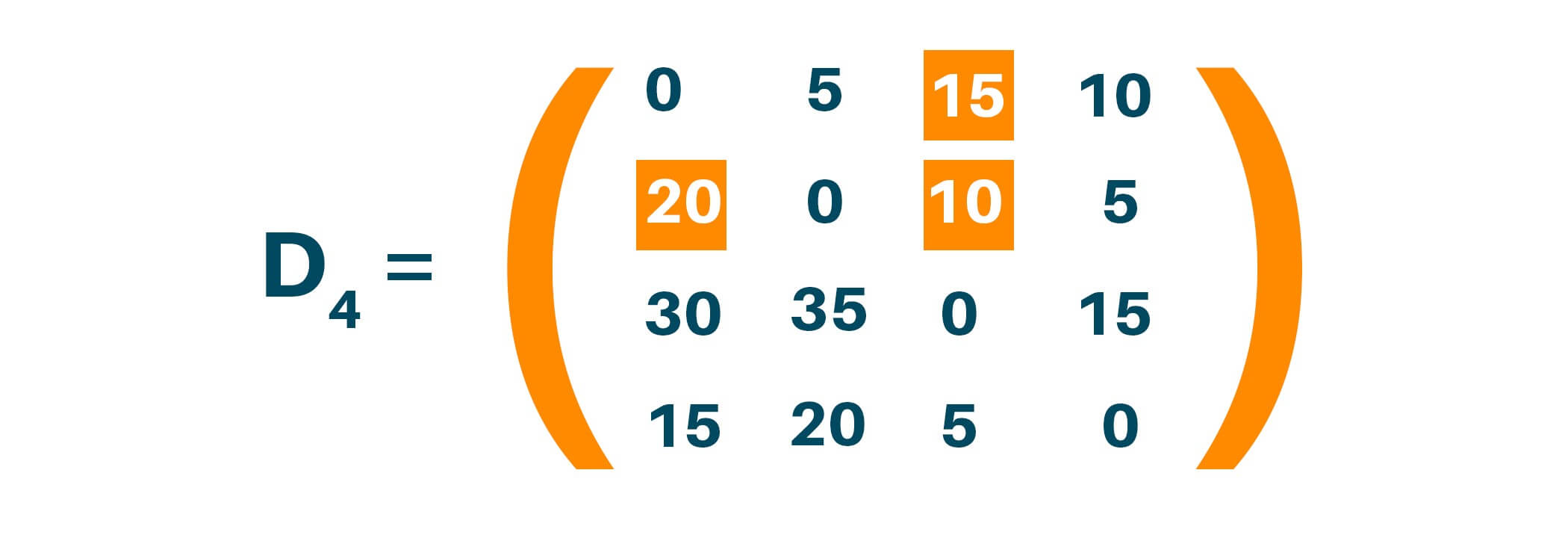## Python Code for Floyd Warshall Algorithm

```# Number of vertices
nV = 4
INF = 999

# Algorithm
def floyd(G):
dist = list(map(lambda p: list(map(lambda q: q, p)), G))

for r in range(nV):
for p in range(nV):
for q in range(nV):
dist[p][q] = min(dist[p][q], dist[p][r] + dist[r][q])
sol(dist)

# Printing the output
def sol(dist):
for p in range(nV):
for q in range(nV):
if(dist[p][q] == INF):
print("INF", end=" ")
else:
print(dist[p][q], end="  ")
print(" ")

G = [[0, 5, INF, INF],
[50, 0, 15, 5],
[30, INF, 0, 15],
[15, INF, 5, 0]]
floyd(G)
```

Output

0  5  15  10

20  0  10  5

30  35  0  15

15  20  5  0

### Time Complexity

There are three loops for computing the shortest path in the graph and each of these loops has constant complexities. Therefore, due to this, the time complexity of the Floyd Warshall algorithm is O(n3). Also, the space complexity of the Floyd Warshall algorithm is O(n2).

### Application of Floyd Warshall Algorithm

• Floyd Warshall Algorithm helps to find the inversion of real matrices
• It helps in testing whether the undirected graph is bipartite
• It helps to find the shortest path in a directed graph
• Different versions of the Floyd Warshall algorithm help to find the transitive closure of a directed graph
• This algorithm helps to find the regular expression the are accepted by finite automata.
• It helps in finding the similarity between the graphs
• Floyd Warshall algorithm helps in finding the optimal routing i.e the maximum flow between two vertices

## Conclusion

Therefore, in the above article, we studied what is Floyd Warshall algorithm and how it is different from Dijkstra's algorithm for finding the shortest path between all vertices in a weighted graph. We studied the algorithm for Floyd Warshall along with the example explaining the algorithm in detail. We learned the python code with its corresponding output and the time complexity to run the algorithm on any weighted graph. Lastly, we understood the application of the Floyd Warshall algorithm which can help us to apply it in real life.

### FavTutor - 24x7 Live Coding Help from Expert Tutors!